Next: 12. The Proof System Up: 2. User's Guide Previous: 10. Quasi-Constructors

Subsections

# 11.1 Motivation

Much of mathematics deals with the study of structures such as monoids, fields, metric spaces, or vector spaces, which consist of an underlying set S, some distinguished constants in S, and one or more functions on S. In IMPS, an individual structure of this kind can be easily formalized as a theory. For example, the IMPS theory for monoids is specified by two def-forms:

   (def-language MONOID-LANGUAGE
(embedded-languages h-o-real-arithmetic)
(base-types uu)
(constants
(e uu)
(** (uu uu uu))))

(def-theory MONOID-THEORY
(component-theories h-o-real-arithmetic)
(language monoid-language)
(axioms
(associative-law-for-multiplication-for-monoids
"forall(z,y,x:uu, x**(y**z)=(x**y)**z)")
("forall(x:uu,x**e=x)")
("forall(x:uu,e**x=x)")))

In the mathematical syntax, the sort uu is written U and the constants e, star* are written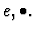Notice that the theory of a monoid as presented here is suitable for concept formulation and proof in a single monoid. Here are some examples:

• One can prove uniqueness of the multiplicative identity: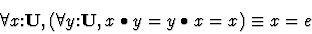• A sequential product operator for sequences can be defined as the least solution of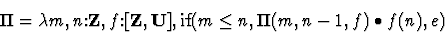• One can easily prove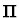satisfies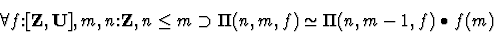• An monoid endomorphism (i.e., a self homomorphism) can be defined by the predicate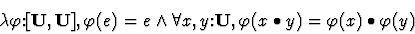However, there is no way to talk about monoid homomorphisms between different monoids.

Nevertheless, it is possible to produce a suitable theory in IMPS in which a general notion of monoid homomorphism can be defined. To do so requires at the very least a theory with two possibly distinct monoids. In general, multiple instances of structures can be dealt with in IMPS in two ways:

• In one approach, we first formalize set theory as an IMPS theory. This can be done by treating Set as a primitive sort and the membership relation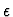as a primitive function symbol. It is then possible to define a structure of a particular kind by a predicate on Set. For instance, a monoid is any object in Set of the form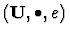where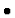is a binary associative operation on U for which e is a right and left identity.
• The other approach is to create a new theory which is the union of embedded replicas of a theory of a single structure. A replica of a theory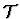is a theory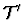, which differs fromonly by an appropriate renaming of sorts and constants. By an embedding we mean a theory interpretation which maps distinct sorts and constants to distinct sorts and constants.
In this section we explain the theory ensemble mechanism, which implements the second approach above. To illustrate how the machinery works in the case of a monoid, let us define a new theory, monoid-theory-1 obtained by renaming the sorts and constants of monoid-theory not in h-o-real-arithmetic by affixing the characters  _1.''
   (def-language MONOID-LANGUAGE-1
(embedded-languages h-o-real-arithmetic)
(base-types uu_1)
(constants
(e_1 uu_1)
(**_1 (uu_1 uu_1 uu_1))))

   (def-theory MONOID-THEORY-1
(component-theories h-o-real-arithmetic)
(language monoid-language-1)
(axioms
(associative-law-for-multiplication-for-monoids
"forall(z,y,x:uu_1,
x **_1 (y **_1 z)=(x **_1 y) **_1 z)")
("forall(x:uu_1,x **_1 e_1=x)")
("forall(x:uu_1,e_1 **_1 x=x)")))

Now define monoid-pair as the union of monoid-theory and monoid-theory-1:
   (def-theory MONOID-PAIR
(component-theories monoid-theory monoid-theory-1))

The theory ensemble facility automates these steps and also provides several bookkeeping devices for keeping track of theories and interpretations in the ensemble.

# 11.2 Basic Concepts

A copy of a theory# 11.3 Usage

There are four def-forms for building and manipulating theory ensembles.
• def-theory-ensemble. This form is used to build a theory ensemble from a given theory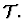The theoryis called the base theory of the ensemble.
• def-theory-ensemble-multiple. This form is used to build a theory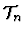which is the union of n copies of• def-theory-ensemble-instances. This form is used to build a theory interpretation from a theory multipleto a given theory.
• def-theory-ensemble-overloadings. This form establishes overloadings for the constants defined inand all the multiples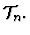# 11.4 Def-theory-ensemble

This is the def-form for building a theory ensemble from an IMPS theory. In the simplest version, the form requires only one argument, the name of the theory ensemble which is then identical to the name of the base theory. For example:
   (def-theory-ensemble METRIC-SPACES)

Evaluating the def-form builds a theory ensemble also named METRIC-SPACES. In general, three additional keyword arguments are allowed:
• (base-theory theory-name). theory-name is the name of the base theory of the ensemble. This keyword arguiment is used when the name of the ensemble is different from that of the base theory.
• (fixed-theories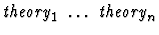. The presence of this argument causes IMPS to rename only those sorts and constants which do not belong to any of the theorieswhen building replicas. The default value of this argument is the fixed-theories set at the time the form is evaluated.
• (replica-renamer proc-name). proc-name is the name of a procedure of one integer argument, used to name sorts and constants of theory replicas. The default procedure is to affix an underscore followed by a nonnegative integer to a name. Most users should be content to use the default.

Another example which utilizes the fixed-theories option is the theory ensemble of vector spaces over a field:
   (def-theory-ensemble VECTOR-SPACES
(fixed-theories fields))


When a theory ensemble def-form is evaluated, IMPS builds a number of tables, all of which are initially empty. The table will be filled as multiples are built. The tables are:

• A table of the theory replicas. The key is an integer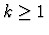and the entry is the k-th replica of the base theory.
• A table of the theory multiples. The key is an integerand the entry is the k-th multiple of the base theory.
• A table of canonical interpretations between multiples. The key is a pair of integers (m, n) with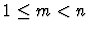and the corresponding entry is a list of interpretations from the m-multiple to the n-multiple.

# 11.5 Def-theory-multiple

This form requires two arguments, the name of a theory ensemble and an integer n. For example,
   (def-theory-ensemble-multiple metric-spaces 2)

When this form is evaluated, IMPS builds a 2-multiple of the theory metric-spaces. IMPS automatically names this theory metric-spaces-2-tuples.

Once the theory metric-spaces-2-tuples is available several important concepts can be defined. For instance,

   (def-constant CONTINUOUS
"lambda(f:[pp_0,pp_1],forall(v:sets[pp_1],
open(v) implies open(inv_image(f,v))))"
(theory metric-spaces-2-tuples))


# 11.6 Def-theory-ensemble-instances

This form is used to build interpretations from selected theory multiples and to transport natively defined constants and sorts from these multiples using these interpretations. The syntax for this def-form is very elaborate, so we refer you to Chapter 17 for its general use.

As an example, suppose we wanted to consider the pair consisting of a normed linear space and the real numbers as a special case of the theory metric-spaces-2-tuples. One reason for doing this is to make all the mathematical machinery developed in the abstract theory metric-spaces-2-tuples available in the particular case. This machinery includes definitions (such as the definition of continuity for mappings) and theorems (such as the various characterizations of continuity.)

We would like to build a theory interpretation from metric-spaces-2-tuples to the union of normed-linear-spaces and h-o-real-arithmetic which is normed-linear-spaces itself, since normed-linear-spaces is a super-theory of h-o-real-arithmetic. Moreover, we would like IMPS to translate all the defined sorts and constants in metric-spaces-2-tuples to normed-linear-spaces.

This can be accomplished by evaluating the form

   (def-theory-ensemble-instances METRIC-SPACES
(target-theories
normed-linear-spaces
h-o-real-arithmetic)
(multiples 1 2)
(sorts (pp uu rr))
(constants
(dist "lambda(x,y:uu, norm(x-y))"
"lambda(x,y:rr, abs(x-y))")))

This does two things: First it builds an interpretation from metric-spaces-2-tuples into normed-linear-spaces in which
• pp_0 goes to uu.
• pp_1 goes to rr.
• dist_0 goes to lambda(x,y:uu, norm(x-y).
• dist_1 goes to lambda(x,y:rr, abs(x-y)).
Secondly, it translates all the natively defined constants in metric-spaces and metric-spaces-2-tuples into normed-linear-spaces.

This form takes the following arguments:
• The name of the theory ensemble.
• Any number of integers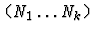Evaluation of the def-form causes IMPS to overload those constants natively defined in the theory multiples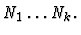For example:
   (def-theory-ensemble-overloadings metric-spaces 1 2)


This form makes the theory ensemble mechanism manageable to the user. When the system transports a natively defined constant c from a theory multiple, it will often give the new constant an unappealing name. For instance, the name might be very long with several indices as suffixes. Ideally, one would like to use the same name for all instances of c, and this is usually possible if the constant is a function symbol which occurs as an operator of an application. In this case, the appropriate function symbol may be unambiguously determined from the sorts of the arguments.

# 11.8 Hints and Cautions

1.
In principle, any theory can be used to build a theory ensemble. However, the theory ensemble facility is useful only if the following two conditions on the base theory are met:
• The base theory is fairly general so that there are likely to be numerous instances of the base theory. Thus it would make little sense to make a theory with essentially only one model into a theory ensemble.
• There is a need for dealing with at least two distinct instances of the base theory.
2.
There is a certain amount of overhead in building theory multiples. This overhead includes building and tabulating theory replicas and building canonical theory interpretations from the m-multiples to n-multiples, where m<n. Recall, that there is a canonical interpretation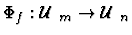for each injective map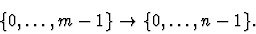There are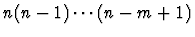such mappings. This overhead can become forbidding when the multiples get too large. As a general rule, multiples of order greater than 4 should be avoided.
3.
Before the system builds an n-multiple it checks whether all j-multiples for j<n already exist, building the missing multiples as needed.Next: 12. The Proof System Up: 2. User's Guide Previous: 10. Quasi-Constructors